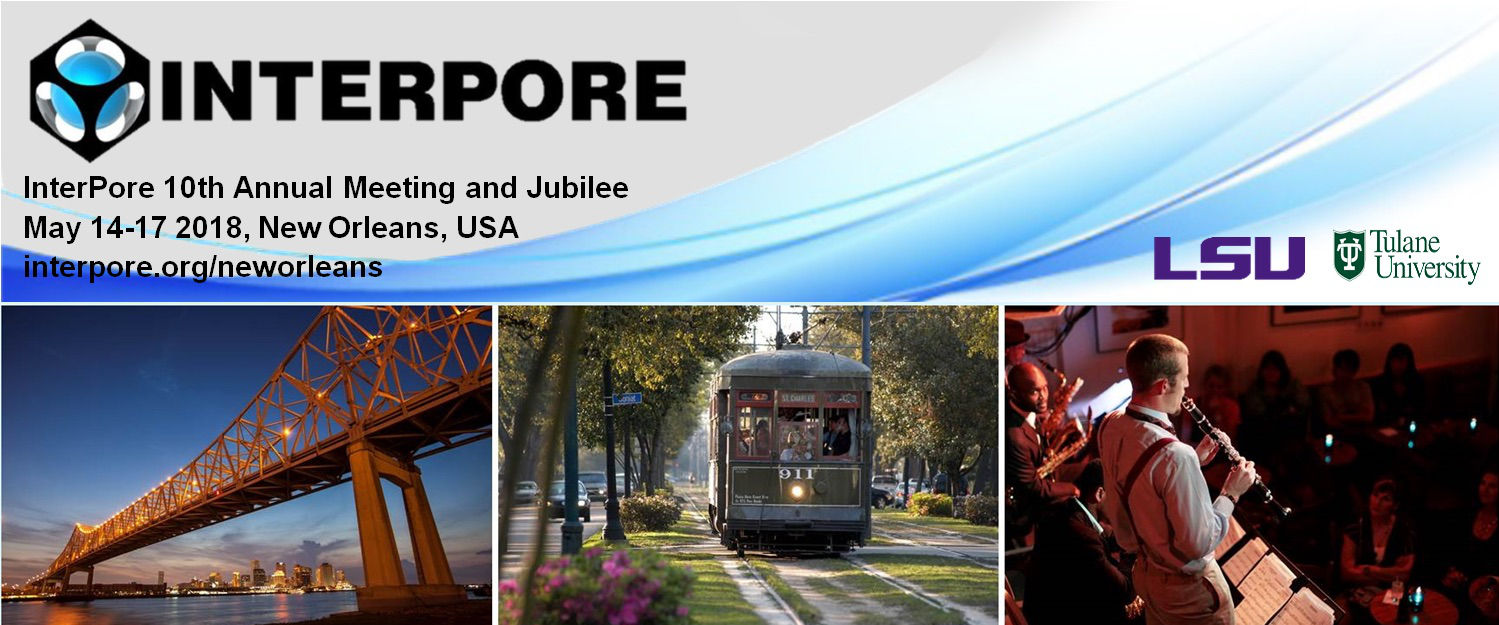#InterPore2018 New Orleans

14-17 May 2018
New Orleans
US/Central timezone

## Theory and Molecular Simulation of Methane Hydrate in Porous Media

17 May 2018, 12:15
1h 30m
New Orleans

#### New Orleans

Poster MS 3.04: Micro and nano fluidic approaches  for studying flow, transport and crystallization processes in porous media

### Speaker

Mr Dongliang Jin (CNRS and Laboratoire Interdisciplinaire de Physique (LIPHY), Universite Grenoble Alpes, F-38000 Grenoble, France)

### Description

Methane hydrate is a non-stoichiometric crystalline structure in which water molecules form hydrogen-bonded cages with methane molecules inside. Abundant methane hydrate resources are present on Earth, especially in various mineral porous media (e.g., permafrost and sea-floor). Methane hydrate is important for many applications in the field of energy and environmental science.[2,3] Understanding the formation, stability, and thermodynamics of methane hydrate in porous media has therefore attracted a great deal of attention in the last decade.

The goal of the present work is to combine computer modeling and theoretical approaches to predict the liquid--hydrate--vapor (L--H--V) phase coexistence of methane hydrate confined in porous media. First, molecular simulation, including molecular dynamics, Monte Carlo simulations, and free energy calculations using the Einstein Molecule approach, is used to determine the chemical potential, free energy, and pressure--temperature ($P$--$T$) phase diagram for bulk methane hydrate (in equilibrium with liquid water and methane vapor). This strategy also provides a mean to determine the corresponding equilibrium methane occupancy, i.e., the methane composition in the hydrate phase. Then, molecular simulation using the direct coexistence method (DCM) is used to determine the pore size effect on melting temperature $T_m$ of methane hydrate. To do so, methane hydrate and liquid water are confined in porous media with various pore size $D_p$. For each $T$ and $P$, the system is set in equilibrium with an infinite reservoir of particles imposing its chemical potential for each species $\mu_m$ and $\mu_w$ (grand canonical ensemble). At a given $P$, the high symmetry phase (hydrate) will be stable below the melting temperature $T_m$ while the low symmetry phase (liquid) will be stable above $T_m$. Our first results suggest that the shift in melting temperature with respect to the bulk, $\Delta T_m = T_{m}^{pore} - T_{m}^{bulk}$, is negative. This is consistent with the Gibbs-Thompson equation with a lower hydrate-wall surface tension than the liquid-wall surface tension, which predicts that $\Delta T/T \sim 1/D_p$. A theoretical derivative for such Gibbs-Thompson equation is analyzed, and free energy calculations using umbrella sampling are used to confirm these findings.

### References

 G. J. MacDonald, Annual Review of Energy, 1990, 15, 53.
 L. J. Florusse, C. J. Peters, J. Schoonman, K. C. Hester, C. A. Koh, S. F. Dec, K. N. Marsh and E. D. Sloan, Science, 2004, 306, 469.
 F. Sch ̈uth, Nature (London), 2005, 434, 712